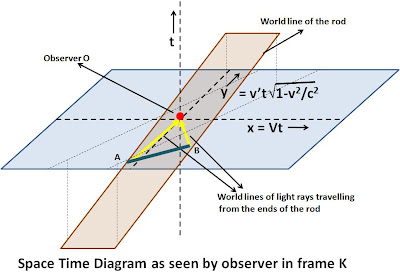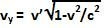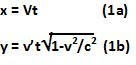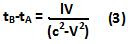## Sunday, December 27, 2009

### Irodov Problem 1.364As seen by observer O' :
Without loss of generalization let us assume that observer O' is at the origin (x'=0,y'=0,t'=0). x',y' and t' being the coordinates in frame K' as seen by O'. As seen by O', the rod moves only along the y' axis at a speed v'. In other words y'=v't'. The rod however, has no motion along the x'-axis. Further assume that the rod is places such that the x' coordinate of its mid point is at x'=0 (this only makes solving the problem easier but does not fundamentally change the result).

At time t'=0, O' sees the two ends of the rod and finds them perfectly parallel to the x'-axis. The ends of the rod A and B as seen by O' are actually due to two different rays of light that started some times in the past t'A and t'B and both reached O' simultaneously at t'=0. Since, the rod is not moving along the x'-axis, and O ' saw the rod to be parallel to the x'-axis, both these times must have been exactly the same i.e. t'A = t'B.

The space-time diagram of the entire situation is depicted in the figure beside. The world-line of the rod is a plane tilted at an angle to the x'y' plane. The world-lines of the ends of the rod only change their y',t' coordinates but not their x' coordinates. The light rays are emitted along the surface of a cone but we depict only those rays that reach the observer O'. These rays of light move at 45 degrees to the x'y' plane but lie entirely in the world-line of the rod.As seen by observer O :
Now lets us see the same phenomenon through the eyes of an observer O located in Frame K at the origin i.e. (x=0,y=0,t=0), where x,y,t are the space time coordinates of frame K. Let us assume that the origins of frames K and K' coincide. As seen by O, the rod moves along the x-axis with a speed V. It also moves long the y-axis with a speed(vy is less than v' because time t' in frame is running slower as seen by O, in other words the same distance along the y-axis is covered in a shorter time when seen in frame O. The same can also be derived the same way as it was derived in Problem 1.358) . In other words, the coordinates of the center of the rod are given by,The world-line of the rod as seen by O in frame K is depicted in the figure. The light rays traveling from the ends of the rod reach the origin at the same time but unlike O', O arrives at different conclusion, namely the light ray that left end A started at a time tA, that is before the time tB, when the ray from end B started its journey. This also means that the end A was a little behind along the y-axis than the end B, in other words the rod was not parallel to x-axis.

Now let us compute the angle the rod is at to the x-axis as seen by O. First we will start by computing the difference tA - tB.

Let the length of the rod as seen in frame K be l. Thus, both the rays of light have to cover a distance of l/2 along the rod.

As we trace back the light ray starting from end A in time, the ray moving at speed c has to "catch up" with the moving rod at it moves at a rate V to the left. This means that,The negative sign comes because, the time tA is in the past to t=0.

The situation is different when we trace back to find out when the ray from rod B started its journey. In this case, the rod is aiding the ray in catching up by moving towards it at a rate V. Hence, we have,Thus, we have,During this time interval tB - tA the end B moved a distance of vy(tB - tA) farther along the y-axis than end A.As shown in the figure thus, we can compute the angle to the x-axis as,